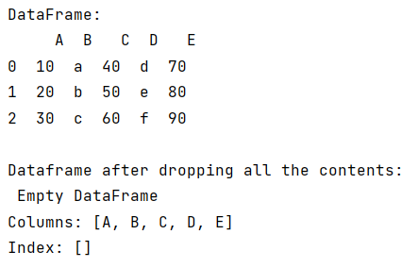# Python - Drop all data in a pandas dataframe

Given a Pandas DataFrame, we need to drop the entire contents of this DataFrame. By Pranit Sharma Last updated : September 25, 2023

Pandas is a special tool that allows us to perform complex manipulations of data effectively and efficiently. Inside pandas, we mostly deal with a dataset in the form of DataFrame. DataFrames are 2-dimensional data structures in pandas. DataFrames consist of rows, columns, and data.

## Problem statement

Given a Pandas DataFrame, we need to drop the entire contents of this DataFrame.

## Dropping all data in a pandas dataframe

To drop all the data in a DataFrame, pandas has a method called pandas.DataFrame.drop() method. It allows us to remove the column according to the column name. This method is used to remove a specified row or column from the pandas DataFrame. Since rows and columns are based on index and axis values respectively, by passing the index or axis value inside pandas.DataFrame.drop() method we can delete that particular row or column. Below is the syntax,

```df.drop(axis=None) # Deletes a specified column
```

To drop all the rows, we will have to pass all the indexes inside pandas.DataFrame.drop(), by using df.index, we can pass the entire row and column structure in this method.

Let us understand with the help of an example,

## Python program to drop all data in a pandas dataframe

```# Importing pandas package
import pandas as pd

# Creating a dictionary
d = {
'A':[10,20,30],
'B':['a','b','c'],
'C':[40,50,60],
'D':['d','e','f'],
'E':[70,80,90]
}

# Creating a dataframe
df = pd.DataFrame(d)

# Display Dataframe
print("DataFrame:\n",df,"\n")

# Droping the Dataframe
df.drop(df.index , inplace=True)

# Display modified DataFrame
print("Dataframe after dropping all the contents:\n",df)
```

### Output

The output of the above program is: